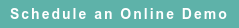# RoyaltyStat Blog

### Posts by Topic

We can test several bivariate (X, Y) regression functions to obtain the most reliable estimate of comparable operating profits. The explanatory variable X can be sales, costs or assets of the selected comparable companies. The dependent variable Y can be sales or operating profits before or after depreciation; and the slope coefficient is an estimate of the comparable operating profit indicator:

(1)     Linear: Y = α + β X, slope = β

(2)     Semilog: Y = α + β LN(X), slope = β / X

(3)     Hyperbola: Y = α – β / X, slope β / X2

(4)     Doublelog: LN(Y) = α + β LN(X), slope = β (Y / X)

(5)     Loghyperbola: LN(Y) = α – β / X, slope = β (Y / X2)

See Goldberger (1964), § 5.2 (Functional Forms), pp. 213-218. We show the slope of (4) on a prior blog: https://blog.royaltystat.com/double-log-operating-profit-margin

We can test these different operating profit functions in RoyaltyStat/Compustat because we have integrated an online regression function with current and historical listed company financials. Thus, we can produce the most reliable estimate of a comparable (arm's length) profit indicator by using the maximum adjusted R2 rule. See Maddala (1977), p. 462. According to the maximum adjusted R2 model selection rule, we can compare models with the same dependent variable, such as we can compare models (1), (2) and (3) or we can compare models (4) and (5), and determine the most reliable profit indicator. The linear model (1) prescribed by the OECD may not produce the most reliable profit indicator under specific facts and circumstances.

In certain economic applications, such the expected relationship between “return on assets” and the risk of its fluctuation (proxied by a robust measure of the standard deviation of “return on assets”), it's customary to postulate a quadratic or parabolic curve. One possible curve specification is the semilog function (2) or the curve:

(6)     Parabolic: Y2 = α + β X,

where we can set Z = Y2 and obtain a linear equation like (1). We see that the estimation of these regression equations can be made after suitable transformation of the X or Y variable. See Maddala (1977), pp. 86-87. [We are considering only bivariate relationships between X and Y, and thus we don't include square or cubic polynomial functions that can produce similar curves as equations (2) or (6)].

Also, instead of selecting a bivariate linear regression function à priori (without first examining a scatterplot of X versus Y to determine the appropriate functional form of the regression model), such as the simplified linear regression model without an intercept promoted by the OECD, we can consider the lessons of Anscombe's quartet. Anscombe (1973) created four datasets that produce identical regression results yet appear different when graphed. Each dataset consists of eleven (X, Y) points that demonstrate the importance of graphing data before analyzing them and the effect of outliers on statistical estimates. Only one of the four datasets produce a good statistical fit using linear model (1).

In sum, even a simple two variables (bivariate compared with multivariate) regression analysis can offer many advantages over a trope calculation of quartiles using the profit ratio β = (Y / X) postulated by the OECD. The purpose of testing these different models of the comparable operating profit function is to obtain more reliable estimates of the slope parameter, and of its accompanying standard error, together with a better statistical fit measured by the maximum adjusted R2.

REFERENCES

Francis Anscombe, “Graphs in Statistical Analysis,” American Statistician, Vol. 27, No. 1 (February 1973). Stable URL: http://www.jstor.org/stable/2682899

Arthur Goldberger, Econometric Theory, John Wiley & Sons, 1964.